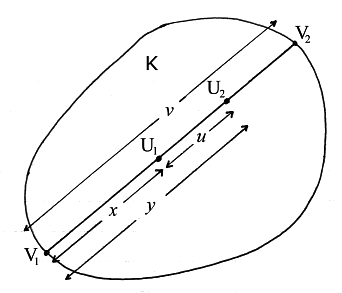PDFs of chord lengths and distances between random points

This webpage is typed up from undated notes (probably 1980s).  Although I haven’t yet found a reference to the main result in the literature, it is unlikely to be original.  MB, January 2014

Fig. 1Let K be a bounded plane convex region (fig. 1).  Let points U1 and U2 have independent uniform distributions inside K.  Let U1U2 produced meet the boundary of K at V1 and V2, where (to avoid ambiguity) V1V2 has azimuth in the range [0,π)

Let U1U2=u with PDF ƒ(u); let V1V2=v with PDF g(v); let V1U1=x and V1U2=y

1.  Given v and x, to find the PDF of y

i.e. to find the probability that y<V1U1<y+δy, where δy is small.

The probability that V1V2=v and V1U1=x exactly is 0; so assume v<V1V2<v+δv and x<V1U1<x+δx, where δv and δx are small compared with δy

Fig. 2Given U1, the permissible set of azimuths of U1U2 will be a (possibly empty) union of small intervals like ε in fig. 2.  (The diagram is drawn for x<y; the change for x>y is straightforward.) The shaded area shows the posssible positions of U2.  Hence

 prob(y1

Thus for 0yv the PDF of y for a given v and x is

 |y−x| . x2−vx+½v2

2.  Given v, to find the PDFs of x and y

Clearly x and y have identical PDFs for a given v; call this PDF φv.  From section 1 we get

 φv(y)= ∫ v |y−x|φv(x)dx . x=0 x2−vx+½v2

Put φv(x)=ψ(x)(x2vx+½v2) and rewrite the last equation as

 (y2−vy+½v2)ψ(y)= ∫ y (y−x)ψ(x)dx− ∫ v (y−x)ψ(x)dx. x=0 x=y

Differentiate twice with respect to y:

 (y2−vy+½v2)ψ′(y)+(2y−v)ψ(y)= ∫ y ψ(x)dx− ∫ v ψ(x)dx, x=0 x=y
 (y2−vy+½v2)ψ″(y)+2(2y−v)ψ′(y)+2ψ(y)=2ψ(y).

Hence

 d [(y2−vy+½v2)2ψ′(y)]=0, dy

i.e., ψ′(y)=A/(y2vy+½v2)2, where A is some constant.  But ψ(y) is symmetrical in the range 0yv; hence ψ′(v/2)=0; hence A=0; hence ψ(y) is a constant.  Since 0vφv(x)dx=1 we conclude

 φv(x)= 3 (x2−vx+½v2). v3

3.  Relation between the PDFs of U1U2 and V1V2

First we find the PDF of u for a given v.  For vuv/2 the PDF is

 (∫ v−u + ∫ v ) uφv(x)dx = 6 u(v−u). 0 u x2−vx+½v2 v3

For 0uv/2 the PDF is

 (∫ u +2 ∫ v−u + ∫ v ) uφv(x)dx = 6 u(v−u) also. 0 u v−u x2−vx+½v2 v3

Hence if u (=U1U2) and v (=V1V2) have respective PDFs ƒ and g then

 ƒ(u)= ∫ ∞ 6 u(v−u)g(v)dv. u v3

Differentiate three times:

 ƒ′(u)= ∫ ∞ 6 u(v−2u)g(v)dv, u v3
 ƒ″(u)=− ∫ ∞ 12 u(v−2u)g(v)dv+ 6 g(u), u v3 u2
 ƒ‴(u)= 12 g(u)− 12 g(u)+ 6 g′(u). u3 u3 u2

On renaming the variable the desired relation can be expressed as:

 t2ƒ‴(t)=6g′(t).

Integration by parts gives

 6g(t)=2ƒ(t)−2tƒ′(t)+t2ƒ″(t),

which can be expressed as:

 g(t)= t3 ⋅ d2 ( ƒ(t) ) . 6 dt2 t

4.  Relation between the moments of U1U2 and V1V2

Let k be a non-negative integer.  If we temporarily define h(t)=ƒ(t)/t, then the last equation gives

 ∫ ∞ tkg(t)dt= 1 ∫ ∞ tk+3h″(t)dt 0 6 0
 = 1 [ tk+3h′(t) ] ∞ − 1 ∫ ∞ (k+3)tk+2h′(t)dt 6 0 6 0
 =0− k+3 ∫ ∞ tk+2h′(t)dt 6 0
 =− k+3 [ tk+2h(t) ] ∞ + k+3 ∫ ∞ (k+2)tk+1h(t)dt 6 0 6 0
 = (k+2)(k+3) ∫ ∞ tkƒ(t)dt. 6 0

Hence the main result of this webpage:

 mean of (V1V2)k= (k+2)(k+3) × mean of (U1U2)k. 6

This result (which has been verified for a rectangle by simulations) is useful in the theory of ley statistics.  For similar results see the discussion in Luis A. Santaló, Integral Geometry and Geometric Probability, 1976, pp. 46–49.